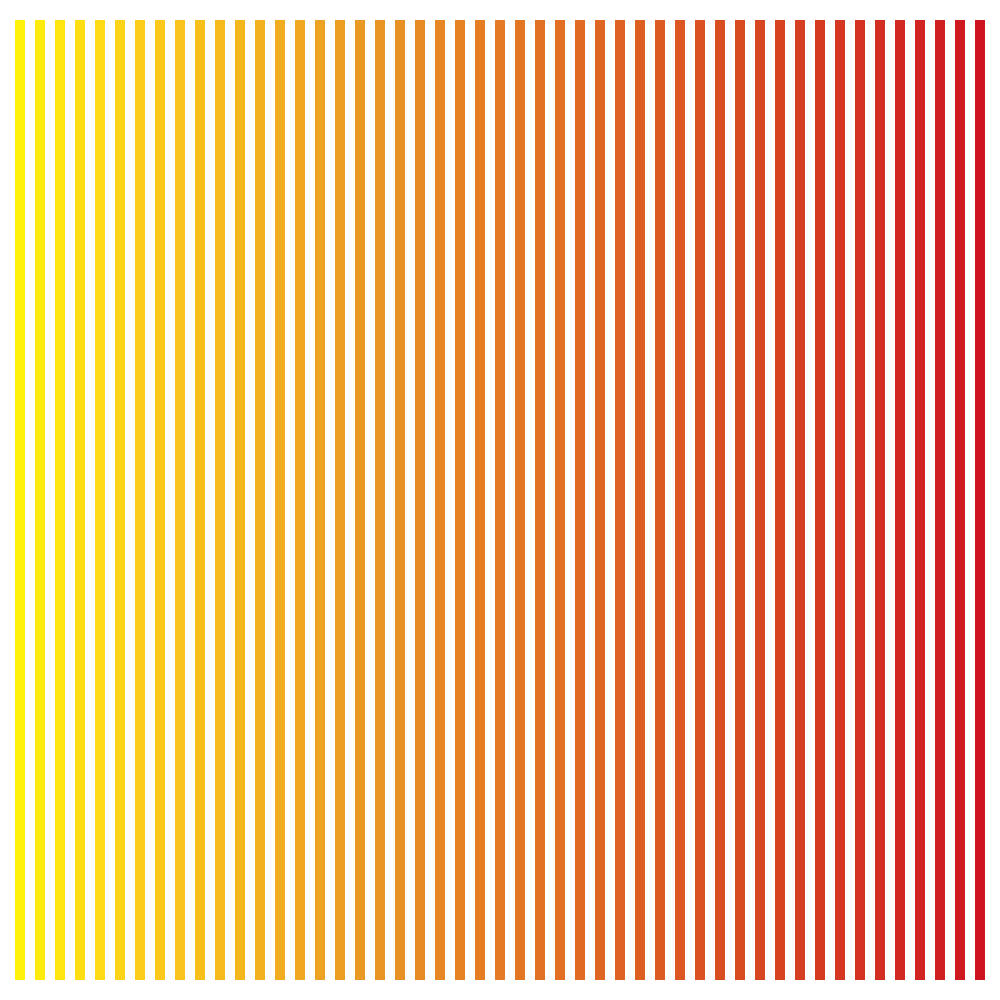# CMYK Stroke¶

Set a stroke before drawing a shape. A cmyk color used while exporting to a .pdf or an image. Handy if the file is used for print.

`cmykStroke`(c, m=None, y=None, k=None, alpha=1)

Set a stroke using a CMYK color before drawing a shape. This is handy if the file is intended for print.

Sets the CMYK stroke color. Each value must be a float between 0.0 and 1.0.

```# define x, y and the amount of lines needed
x, y = 20, 20
lines = 49
# calculate the smallest step
colorStep = 1.00 / lines
# set stroke width
strokeWidth(10)
# start a loop
for i in range(lines):
# set a cmyk color
# the magenta value is calculated
cmykStroke(0, i * colorStep, 1, 0)
# draw a line
line((x, y), (x, y + 960))
# translate the canvas
translate(20, 0)
```